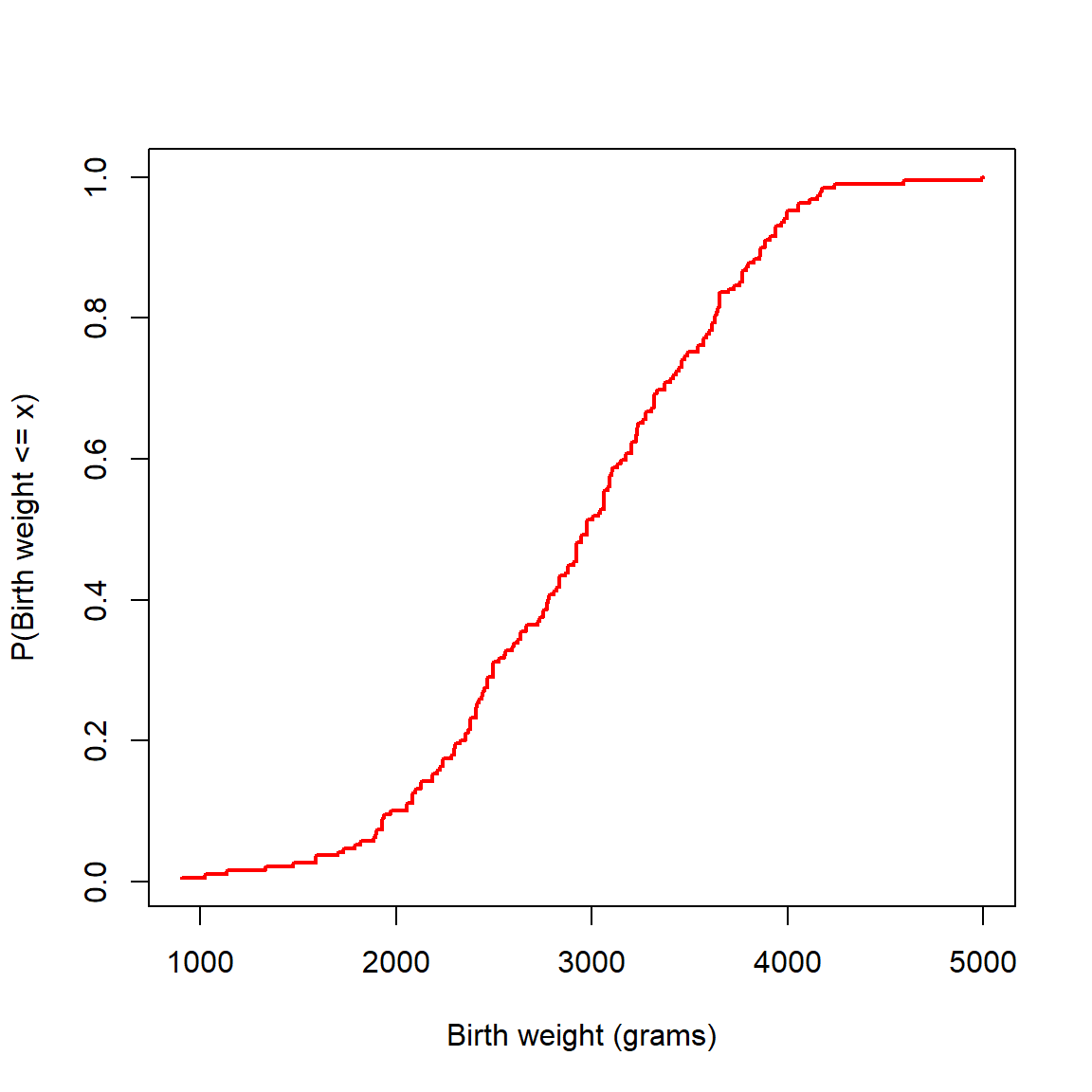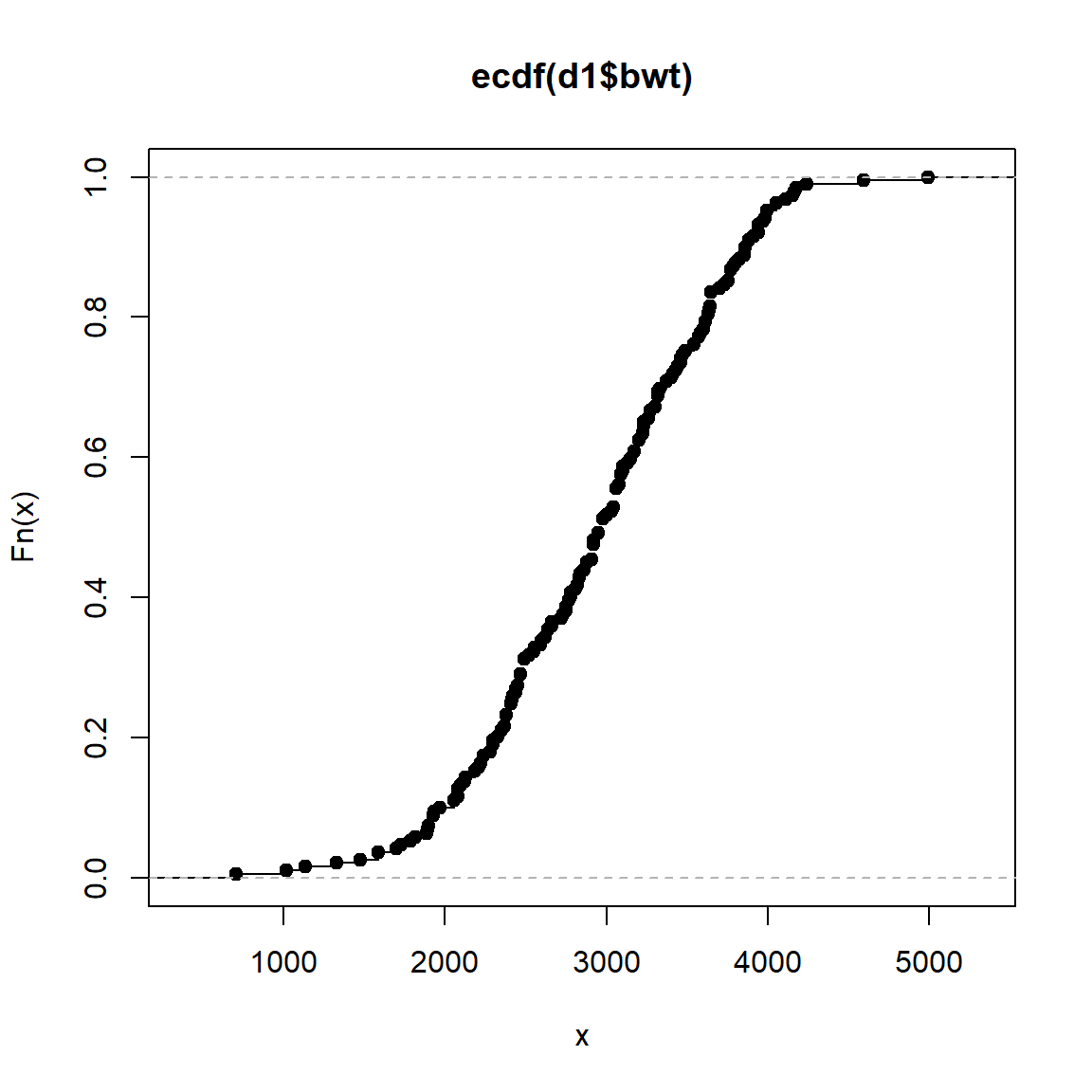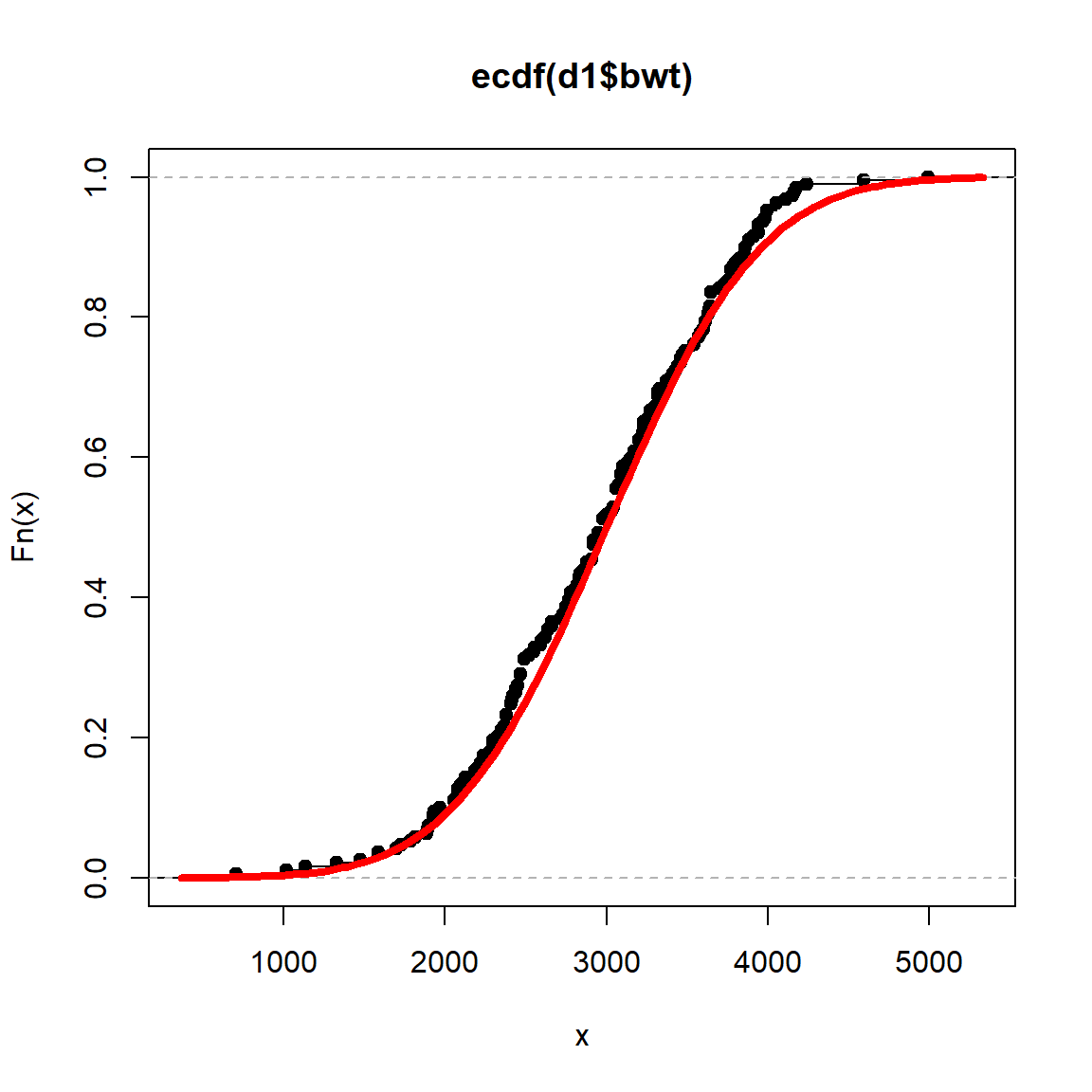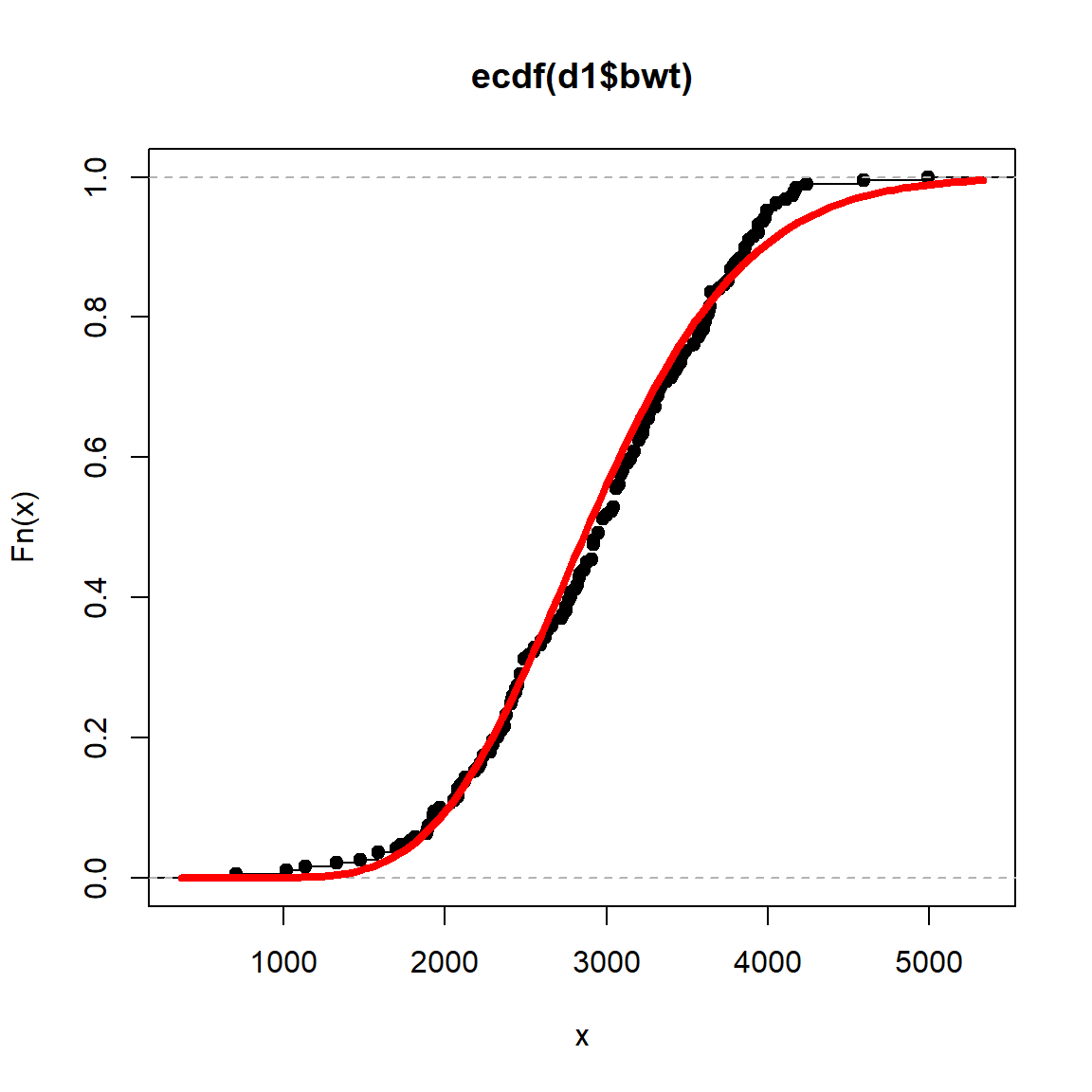# CDFs, PDFs, and Estimation

The birthwt dataset in the MASS package contains 10 measurements of infant-mother pairs, collected in Springfield, MA in 1986. See ?MASS::birthwt for additional details.

Consider the random variable birth weight which is labelled bwt in the dataset and is measured in grams. The distribution of birthweight is uncertain. Neither the distribution function (CDF) or the density function (PDF) are known.

The data collected in the birthwt dataset can be used to estimate the distribution. Let’s first plot the data. There are several ways to plot the data, here we consider the eCDF and the histogram.

## eCDF

Recall that the CDF is a function with takes candidate outcomes as inputs and returns cumulative left hand probabilities as outputs. Let $$F$$ be the distribution function or CDF for infant birthweight.

$F(x) = P(\text{birth weight}\leq x)$

If one were interested in calculating the probability of a birthweight less than or equal to 2000 grams, one could plug 2000 into $$F$$.

$F(2000) = P(\text{birth weight} \leq 2000)$

If the distribution function $$F$$ is unknown, how might one use the dataset to estimate $$F(2000)$$? One approach is to calculate the empirical probability. That is,

$\hat{F}(2000) = \frac{\text{# birth weights less than or equal to 2000}}{\text{# infants in dataset}}$

The hat notation, $$\hat{\ }$$, indicates an estimate of the true, underlying expression. In this case, $$\hat{F}$$ is an estimate of $$F$$, the distribution function of infant birthweight.

In words, the empirical probability is the proportion of observations in the dataset which qualify as the event of interest. In R, we can calculate the proportion with the following command.

d1 <- MASS::birthwt
mean(d1$bwt <= 2000) ##  0.1005291 To generate all cumulative left hand probabilities, we can create a function. The function Fhat is created so that an input a vector x returns an output vector of the same size. Fhat <- function(x,b) colMeans(outer(b, x, "<=")) Fhat(1000, d1$bwt)
##  0.005291005
Fhat(2000, d1$bwt) ##  0.1005291 Fhat(3000, d1$bwt)
##  0.5132275
Fhat(1:4 * 1000, d1$bwt) ##  0.005291005 0.100529101 0.513227513 0.952380952 We can plot Fhat. xxx <- seq(900,5000,by=1) plot(xxx,Fhat(xxx,d1$bwt), col = "red", lwd = 2, type = "l", xlab = "Birth weight (grams)", ylab = "P(Birth weight <= x)")Note that Fhat has all the properties of a CDF. It starts at zero, goes to one, and is non-decreasing. Because this estimate of the distribution function is so important, it has its own name and command. Fhat is known as the empirical cumulative distribution function or eCDF. In R, one can generate the eCDF from a vector using the ecdf command.

ecdf(d1$bwt) ## Empirical CDF ## Call: ecdf(d1$bwt)
##  x[1:131] =    709,   1021,   1135,  ...,   4593,   4990
plot(ecdf(d1$bwt))Assessment 1. Create a plot of the eCDF of mother’s age in years. 2. Based on the eCDF, what is the probability of observing an age of 35 or higher among mother-infant pairs? ## Smoothing The eCDF is a powerful tool, but it has its drawbacks. An eCDF is not smooth. In many instances, the true underlying distribution is believed to be smooth. There are a number of ways to estimate the distribution so that the resulting estimate is smooth. For example, one may 1. Choose from a smooth family of distributions 2. Choose from a mixture of smooth distributions 3. Use a smoothing kernel ### Smooth families There are several well known and widely used distributions which may describe the distribution of birth weights. Navigate to this Desmos calculator (link) You’ll see the eCDF of the birth weight data. #### Gaussian or Normal or Bell distribution Click the circle in line 5. This will display the distribution function of a Gaussian or Normal random variable. This distribution as two parameters: the mean and standard deviation. Line 3 is a slider that controls the mean. Line 4 has a slider that controls the standard deviation. Assessment: 1. Use the sliders to find a Gaussian distribution which describes the birth weight data reasonably well. 2. What are your values of the mean and standard deviation? 3. How does the mean parameter change the shape of the distribution? How about the standard deviation parameter? The mathematical expression for the Gaussian distribution is not simple. If $$\mu$$ is the mean and $$\sigma$$ is the standard deviation, then $F(x) = \int_{-\infty}^x (2\pi\sigma^2)^{-\frac{1}{2}} \exp\left(-\frac{1}{2}\frac{(t-\mu)^2}{\sigma^2} \right)\, dt$ Because the CDF of the Gaussian distribution is so important, it is generally denoted with the expression $$\Phi(x, \mu, \sigma^2)$$. In R, the Gaussian distribution function is pnorm(x, µ, σ). To plot the Gaussian CDF with $$\mu=$$ 3000 and $$\sigma=$$ 750 overlaid on the eCDF, use pnorm(x, 3000, 750), as in the code below. plot(ecdf(d1$bwt))
curve(pnorm(x,3000,750), add=TRUE, col = "red", lwd = 4)Assessment:

1. Generate a plot of the Gaussian CDF overlaid on the eCDF using the parameters you identified in the previous assessment.
2. The term low birth weight refers to infants which weight less than 2500 grams. Based on your Gaussian model of birthweight (with parameters you selected by eye), what is the probability of observing a low birth weight infant?
3. Create a plot of the eCDF of mother’s age in years. Overlay the Gaussian CDF with the sample mean (mean(d1$age)) and sample standard deviation (sd(d1$age)) of age as the parameters.

#### Gamma distribution

Click the circle in line 5 again (to make it disappear). Now click line 10 to reveal the CDF of the gamma distribution. This distribution also has two parameters. (Sometime the parameters are shape and scale and in other instances they are shape and rate. Here, we use shape ($$\alpha$$) and rate ($$\beta$$).) The lines 6 and 7 control the parameters for the gamma distribution.

Assessment:

1. Use the sliders to find a gamma distribution which describes the birth weight data reasonably well.
2. What are your values of $$\alpha$$ and $$\beta$$?

In R, the gamma distribution function is pgamma(x, α, β). To plot the gamma CDF with $$\alpha=$$ 14.75 and $$\beta=$$ 0.005 overlaid on the eCDF, use pgamma(x, 14.75, 0.005), as in the code below.

plot(ecdf(d1$bwt)) curve(pgamma(x,14.75,0.005), add=TRUE, col = "red", lwd = 4)Assessment: 1. Generate a plot of the gamma CDF overlaid on the eCDF using the parameters you identified in the previous assessment. 2. The term very low birth weight refers to infants which weight less than 1500 grams. Based on your gamma model of birthweight (with parameters you selected by eye), what is the probability of observing a very low birth weight infant? 3. Create a plot of the eCDF of mother’s age in years. Overlay the gamma CDF with $$\alpha=$$mean(d1$age)^2/var(d1$age) and $$\beta=$$mean(d1$age)/var(d1\$age) as the parameters.

### Mixtures of smooth distributions

Another way to estimate a smooth distribution is to take a weighted average of two or more smooth distributions. For example, one could take the average of two smooth Gaussian distribution functions. There would be 5 parameters: $$\mu$$ and $$\sigma$$ for the first distribution, $$\mu$$ and $$\sigma$$ for the second distribution, and $$\alpha$$ a number between 0 and 1 which indicates the relative weight of the first distribution to the second.

Mathematically, the mixture of 2 normals is expressed as:

$\hat{F}(x) = \alpha \Phi(x, \mu_1, \sigma_1) + (1-\alpha) \Phi(x, \mu_2, \sigma_2)$

If $$\alpha=1$$ then the CDF would be the first distribution, $$\Phi(x, \mu_1, \sigma_1)$$. If $$\alpha = 0$$, then the CDF would be the second distribution, $$\Phi(x, \mu_2, \sigma_2)$$. Mixture distributions admit a larger number of possible functions than the individual components alone.

Click the circle in line 10 again (to make it disappear). Now click line 16 to reveal the CDF of the mixture of 2 Gaussian distributions. The lines 11 to 15 control the parameters for the mixture distribution.

Assessment:

1. Use the sliders to find a mixture distribution which describes the birth weight data reasonably well.
2. What are your values of the parameters?
3. Of all the distributions in this module, which estimate of the CDF best describes birth weight in your opinion?
4. How did you judge which estimate was best? What was your criteria?
5. Create a plot of the eCDF of mother’s age in years. Overlay the CDF of the mixture of 2 Gaussians with $$\mu_1=$$ 19.8, $$\sigma_1=$$ 2.8, $$\mu_2=$$ 26.4, $$\sigma_2=$$ 5, and $$\alpha=$$ 0.48.
6. Among the Gaussian, gamma, and mixture distributions, which estimate gave the best description of the distribution of maternal age, in your opinion? Explain why.

### Kernel methods for estimation of smooth distributions

This section will be covered in a later module.